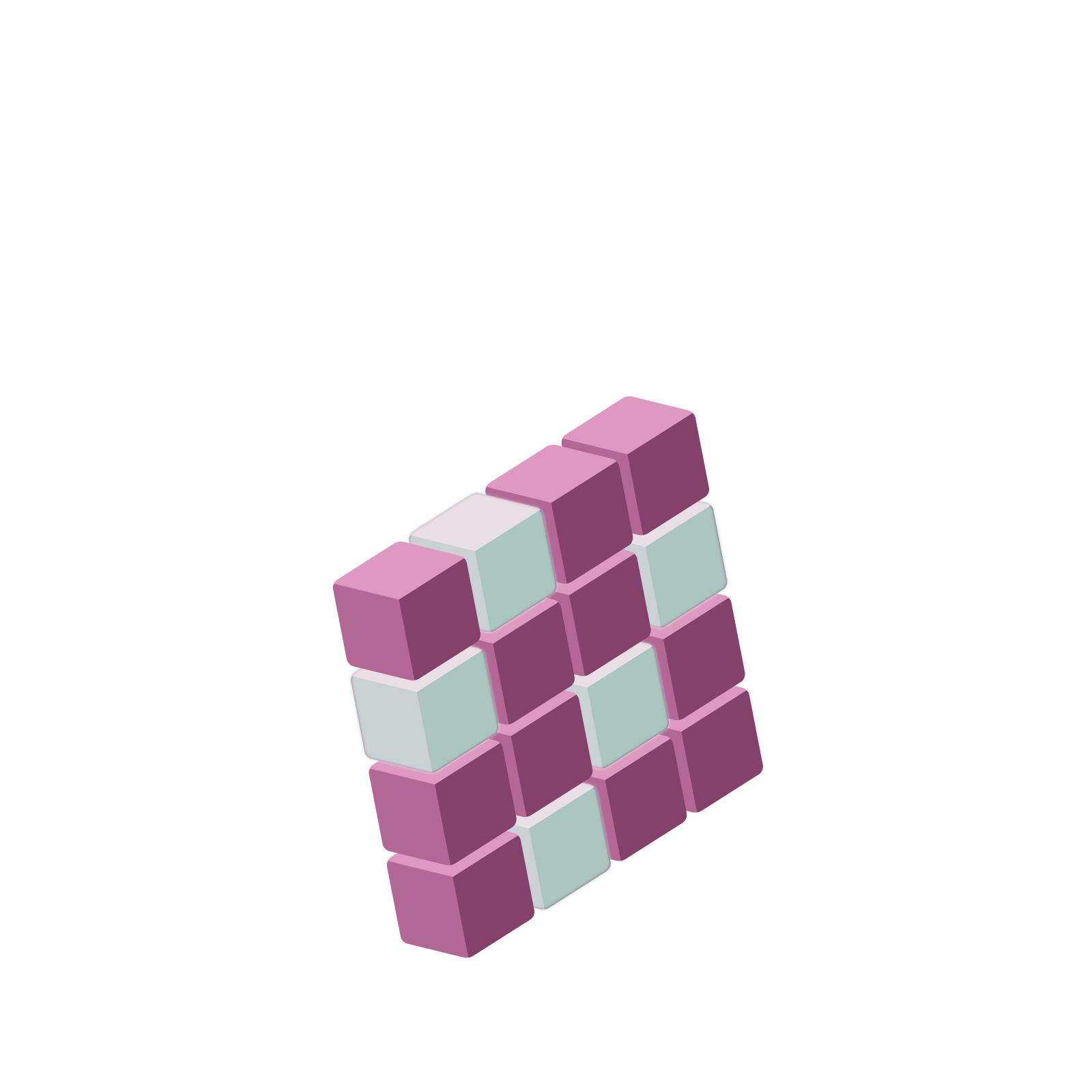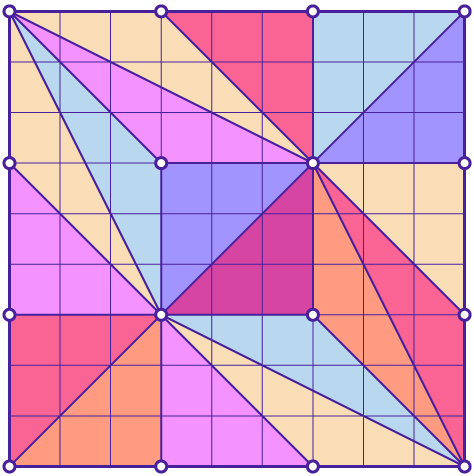06 103 624# Station  10EWho Really Cares about Ten-adic and other “adic” Number Systems?If one conducts an internet search on the topic of “p-adic numbers” (here “p” stands for prime) one will see the extent to which mathematicians care about these number systems. As I mentioned before, they seem to provide a powerful tool for analyzing multiplicative properties of numbers in basic number theory: the properties of prime numbers and prime factorizations of numbers. So many basic questions about numbers and basic arithmetic are still unanswered!

But perhaps the most surprising application of p-adic numbers I’ve seen is an application to geometry.Consider a square. It is possible to subdivide a square into $18$ triangles of equal area.It is fun to find ways to divide a square into $6$ triangles of equal area, or $14$, or $200$. But here’s the shocker.

It is impossible to divide a square into an odd number of triangles of equal area!

This result was proved in 1970 by mathematician Paul Monsky (“On Dividing a Square into Triangles”. The American Mathematical Monthly. 77 (2): 161–164) and he had to use the $2$-adic number system to do so!

One can avoid $2$-adic numbers if one restricts the vertices of the triangles to grid points (as for the diagram above) and my attempt of explaining the proof in this case is here. But I know of no proof of Monsky’s result for the general case without using the $2$-adic number system.

Mathematics, no matter how abstruse as it might seem, always seems to come up with surprising and unexpected applications.